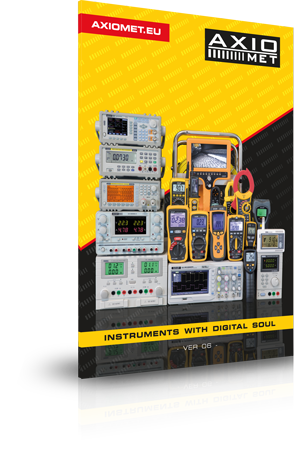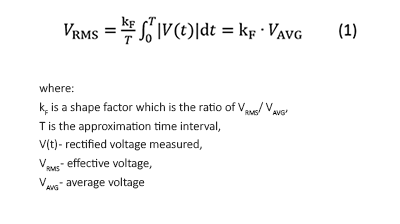Country: United Kingdom
Categories

### AXIOMET Catalogue(ver. 6)
PDF (12,9 MB)

# Measuring alternating signals with the use of True RMS multimeters

You can measure alternating voltage and current with the use of almost all digital multimeters available on the market. However, not all of these devices let you measure the True Root Mean Square value, known as True RMS. Multimeters that are not equipped with a True RMS converter measure the effective value of the signal by approximating a square waveform that they can ‘see’ after sampling. Then, this signal is scaled to effective value according to the following formula: (1):Regardless of the input signal shape, the shape factor value used in order to determine the effective (RMS) value is 1.11 and it corresponds to the ratio of a sinusoidal waveform. It means that the RMS value measurement is the most accurate for sinusoidal signals only.

Measurements of other alternating signals will be imprecise due to different values of kF shape factors. For example, a square wave with a 50% duty cycle will be affected by an 11% error because kF =1.00.

It means that it is possible to accurately measure only sinusoidal alternating voltage. Multimeters without the True RMS feature can be used to measure only mains power which is usually sinusoidal in shape. Measurements of current done with the use of such a multimeter may be inaccurate because the current flowing through the receivers connected to the mains electricity supply is not (and does not have to be) always sinusoidal in shape.

In order to measure current and voltage True RMS values of alternating, non-sinusoidal signals precisely, it is necessary to use a True RMS multimeter.

The main limitation connected with the use of a True RMS multimeter is the speed of signal change which is expressed through:

• max frequency,
• signal rise time
• or the width of the frequency band.

There are numerous situations in which it is wise or even necessary to use True RMS multimeters because we are dealing with alternating, non-sinusoidal signals.

In electronics world we often encounter periodic rectangular, triangular, sawtooth, non-sinusoidal and diversely modulated signals. In order to measure the True RMS values of these signals precisely, it is necessary to use a True RMS multimeter.

Very often, the mains current in a load is deformed and non-sinusoidal due to non-linear character of the receivers (e.g. bulbs).

As far as constant voltage systems are concerned, it is very popular to control the power with the use of pulse width modulators (e.g. controlling the speed of cooling fans used in computers and power supply units). If this is the case, the patterns of the load current and the supply voltage are impulsive in shape (their waveform can be e.g. rectangular or arbitrary), and in order to measure the True RMS value, it is necessary to use a True RMS multimeter.

We have a similar problem with sinusoidal voltage supply systems, in which thyristors and triacs are used for control (e.g. light dimmers). The load current flows in very short time intervals because the supply voltage is switched on by only a part of the mains sine wave.

Another typical example can be measurement of the True RMS value of output current in switched-mode power supplies**, which are widely used in computers, monitors, chargers, and many other household appliances. The pattern of the load current signal is periodic, however, it is impulsive, it has a short rise time, and it lasts for a short period of time. It means that the True RMS value of the current consumed by a lot of modern devices can be measured with True RMS multimeters only.

True RMS multimeters can also be used to determine the active power of a receiver, if it is resistive in nature. We have to measure the effective voltage on the load (VRMS) and the current (IRMS) flowing through this load; then, we have to multiply the results of our measurements. As you can see, thanks to the use of True RMS multimeters we can not only measure supply voltage and load current precisely, but also determine the active power of receivers with switched-mode and alternating voltage power supplies .

To sum up, multimeters with a built-in True RMS converter ensure accurate measurements of the majority of alternating voltage and current signals met in practice. A noticeably higher functionality and accuracy of multimeters with the True RMS feature justifies a slightly higher cost of such measuring devices. Therefore, these multimeters are dedicated to anybody who needs a functional and accurate multimeter for a wide range of applications.

#### Check the offer >>